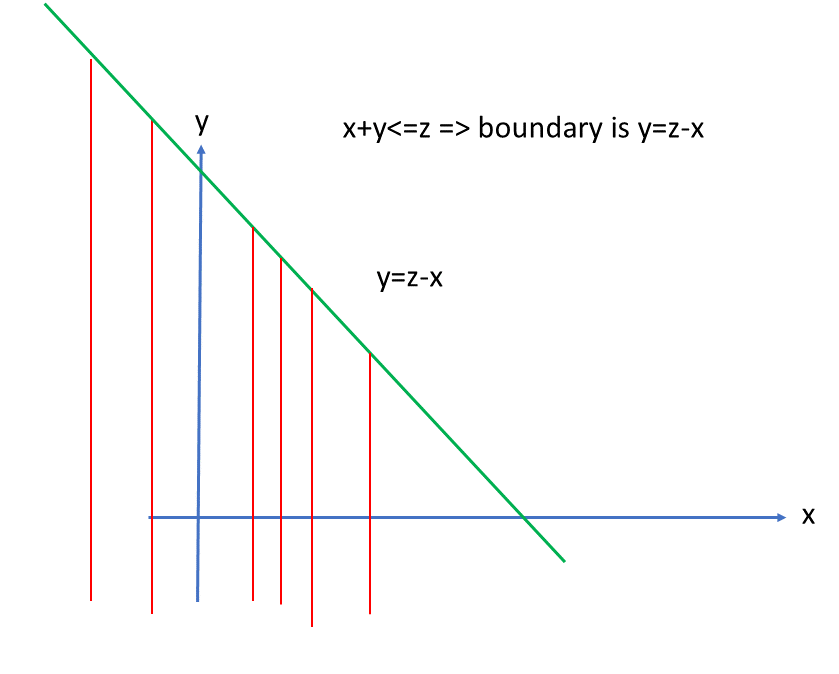14
Dec 21

## Sum of random variables and convolution

### Link between double and iterated integrals

Why do we need this link? For simplicity consider the rectangle$A=\left\{ a\leq x\leq b,c\leq y\leq d\right\} .$ The integrals$I_{1}=\underset{A}{\int \int }f(x,y)dydx$

and$I_{2}=\int_{a}^{b}\left( \int_{c}^{d}f(x,y)dy\right) dx$

both are taken over the rectangle$A$ but they are not the same.$I_{1}$ is a double (two-dimensional) integral, meaning that its definition uses elementary areas, while$I_{2}$ is an iterated integral, where each of the one-dimensional integrals uses elementary segments. To make sense of this, you need to consult an advanced text in calculus. The  difference notwithstanding, in good cases their values are the same. Putting aside the question of what is a "good case", we  concentrate on geometry: how a double integral can be expressed as an iterated integral.

It is enough to understand the idea in case of an oval$A$ on the plane. Let$y=l\left( x\right)$ be the function that describes the lower boundary of the oval and let$y=u\left( x\right)$ be the function that describes the upper part. Further, let the vertical lines$x=m$ and$x=M$ be the minimum and maximum values of$x$ in the oval (see Chart 1).Chart 1. The boundary of the oval above the green line is described by u(x) and below - by l(x)

We can paint the oval with strokes along red lines from$y=l\left( x\right)$ to$y=u\left(x\right) .$ If we do this for all$x\in \left[ m,M\right] ,$ we'll have painted the whole oval. This corresponds to the representation of$A$ as the union of segments$\left\{ y:l\left( x\right) \leq y\leq u\left( x\right) \right\}$ with$x\in \left[ m,M\right] :$$A=\bigcup\limits_{m\leq x\leq M}\left\{ y:l\left( x\right) \leq y\leq u\left( x\right) \right\}$

and to the equality of integrals

(double integral)$\underset{A}{\int \int }f(s,t)dsdt=\int_{m}^{M}\left(\int_{l\left( x\right) }^{u(x)}f(x,y)dy\right) dx$ (iterated integral)

### Density of a sum of two variables

Assumption 1 Suppose the random vector$\left( X,Y\right)$ has a density$f_{X,Y}$ and define$Z=X+Y$ (unlike the convolution theorem below, here$X,Y$ don't have to be independent).

From the definitions of the distribution function$F_{Z}\left( z\right)=P\left( Z\leq z\right)$ and probability$P\left( A\right) =\underset{A}{\int \int }f_{X,Y}(x,y)dxdy$

we have$F_{Z}\left( z\right) =P\left( Z\leq z\right) =P\left( X+Y\leq z\right) =\underset{x+y\leq z}{\int \int }f_{X,Y}(x,y)dxdy.$

The integral on the right is a double integral. The painting analogy (see Chart 2)Chart 2. Integration for sum of two variables

suggests that$\left\{ (x,y)\in R^{2}:x+y\leq z\right\} =\bigcup\limits_{-\infty

Hence,$\int_{-\infty }^{z}f_{Z}\left( z\right) dz=F_{Z}\left( z\right)=\int_{R}\left( \int_{-\infty }^{z-x}f_{X,Y}(x,y)dy\right) dx.$

Differentiating both sides with respect to$z$ we get$f_{Z}\left( z\right) =\int_{R}f_{X,Y}(x,z-x)dx.$

If we start with the inner integral that is with respect to$x$ and the outer integral$-$ with respect to$y,$ then similarly$f_{Z}\left( z\right) =\int_{R}f_{X,Y}(z-y,y)dy.$

Exercise. Suppose the random vector$\left( X,Y\right)$ has a density$f_{X,Y}$ and define$Z=X-Y.$ Find$f_{Z}.$ Hint: review my post on Leibniz integral rule.

### Convolution theorem

In addition to Assumption 1, let$X,Y$ be independent. Then$f_{X,Y}(x,y)=f_{X}(x)f_{Y}\left( y\right)$ and the above formula gives$f_{Z}\left( z\right) =\int_{R}f_{X}(x)f_{Y}\left( z-x\right) dx.$

This is denoted as$\left( f_{X}\ast f_{Y}\right) \left( z\right)$ and called a convolution.

The following may help to understand this formula. The function$g(x)=f_{Y}\left( -x\right)$ is a density (it is non-negative and integrates to 1). Its graph is a mirror image of that of$f_{Y}$ with respect to the vertical axis. The function$h_{z}(x)=f_{Y}\left( z-x\right)$ is a shift of$g$ by$z$ along the horizontal axis. For fixed$z,$ it is also a density. Thus in the definition of convolution we integrate the product of two densities$f_{X}(x)f_{Y}\left( z-x\right) .$ Further, to understand the asymptotic behavior of$\left( f_{X}\ast f_{Y}\right) \left( z\right)$ when$\left\vert z\right\vert \rightarrow \infty$ imagine two bell-shaped densities$f_{X}(x)$ and$f_{Y}\left( z-x\right) .$ When$z$ goes to, say, infinity, the humps of those densities are spread apart more and more. The hump of one of them gets multiplied by small values of the other. That's why$\left(f_{X}\ast f_{Y}\right) \left( z\right)$ goes to zero, in a certain sense.

The convolution of two densities is always a density because it is non-negative and integrates to one:$\int_{R}f_{Z}\left( z\right) dz=\int_{R}\left( \int_{R}f_{X}(x)f_{Y}\left(z-x\right) dx\right) dz=\int_{R}f_{X}(x)\left( \int_{R}f_{Y}\left(z-x\right) dz\right) dx$

Replacing$z-x=y$ in the inner integral we see that this is$\int_{R}f_{X}(x)dx\int_{R}f_{Y}\left( y\right) dy=1.$